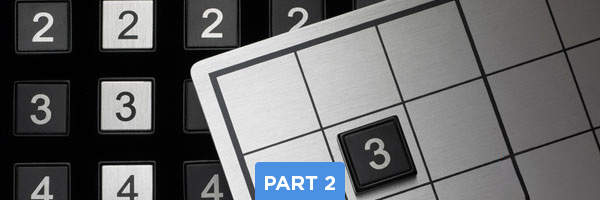# Intermediate Sudoku Solving Techniques – Part 2: Three Rows at a TimeIn this Sudoku grid, we’ll start by looking at the top set of squares – outlined in red. Looking in these top three rows, we can see two number 1s – in the top row and bottom row (circled in blue). There currently is no 1 in the upper left square, but this shows us that the 1 in that square cannot be in the top or bottom row (marked with Xs). Looking to the left set of squares, we can see two more 1s: in the left center square (bottom left space) and the bottom left square (bottom center space), both circled in blue. This helps eliminate more spaces from the top left square, leaving only the left center space as the place where the 1 must go (highlighted in orange).

After placing that number 1, we can keep looking at the top three rows (top set of squares) to try to work through the rest of the numbers, 2-9. We can see that there are already three 2s in place (circled in red) so we can skip #2 – that one is done. These top three rows do not have any 3s, 4s, or 5s, so we can move on to number 6. We can see two 6s – one in the top row, and one in the middle row (circled in blue). By extending the “reach” of those numbers into the middle square, we can eliminate 4 empty spaces which cannot contain number 6 (marked with Xs) so that means, the number 6 must go in the lower left corner (highlighted in orange).

Now that the number 6 has been placed, we can look at the remaining numbers in these top three rows. There are no 7s in these three rows, so we can ignore that number, and there are already three 9s in place (circled in red), so let’s focus on the number 8.

There are two 8s in these top three rows – one in the top row, one in the center row (circled in blue). We need to find out where to place an 8 within the upper left square. By extending the “reach” of those number 8s into the upper left square (outlined in blue) we can eliminate three spaces (marked with Xs). Also, when we look below the upper left square, we can see two other 8s – left center square, center column, and bottom left square, lower-right space (circled in blue). This helps us eliminate another space in the upper left square, meaning that there is only one possible space where the 8 can be within that upper left square: the lower-right space (highlighted in orange).

Now we’ve completed the top three rows in terms of using the “three rows” technique – we’ve worked through all 9 numbers. But you can use this technique to focus on the remaining two sets of three rows – working through numbers 1-9, one at a time, until you’ve eliminated the possibilities. This technique gives you a way to stay focused on one part of the grid at a time, methodically moving forward to find solutions to Sudoku puzzles.

What are your thoughts? Have you ever tried focusing on three rows at a time? How did it work for you? Leave a comment and let us know.